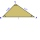# Garden

Area of a square garden is 6/4 of triangle garden with sides 56 m, 35 m, and 35 m.

How many meters of fencing need to fence a square garden?

Result

x =  119 m

#### Solution:Leave us a comment of example and its solution (i.e. if it is still somewhat unclear...):Be the first to comment!#### To solve this verbal math problem are needed these knowledge from mathematics:

Do you have a linear equation or system of equations and looking for its solution? Or do you have quadratic equation? Pythagorean theorem is the base for the right triangle calculator. Do you want to convert length units? See also our trigonometric triangle calculator.

## Next similar examples:

1. Axial sectionAxial section of the cone is an equilateral triangle with area 208 dm2. Calculate the volume of the cone.
2. Triangle SASCalculate the area and perimeter of the triangle, if the two sides are 51 cm and 110 cm long and angle them clamped is 130 °.
3. Tetrahedral pyramidWhat is the surface of a regular tetrahedral (four-sided) pyramid if the base edge a=7 and height v=6?
4. SquarePoints A[-5,-6] and B[7,-1] are adjacent vertices of the square ABCD. Calculate the area of the square ABCD.
5. CubesOne cube is inscribed sphere and the other one described. Calculate difference of volumes of cubes, if the difference of surfaces in 257 mm2.
6. Three altitudesA triangle with altitudes 4; 5 and 6 cm is given. Calculate the lengths of all medians and all sides in a triangle.
7. Trapezoid MOThe rectangular trapezoid ABCD with right angle at point B, |AC| = 12, |CD| = 8, diagonals are perpendicular to each other. Calculate the perimeter and area of ​​the trapezoid.
8. Cone A2VSurface of cone in the plane is a circular arc with central angle of 126° and area 415 cm2. Calculate the volume of a cone.
9. Isosceles triangle 9Given an isosceles triangle ABC where AB= AC. The perimeter is 64cm and altitude is 24cm. Find the area of the isosceles triangle
10. Right ΔA right triangle has the length of one leg 28 cm and length of the hypotenuse 53 cm. Calculate the height of the triangle.
11. Is right?Is triangle with sides 51, 56 and 77 right triangle?
12. CuboidCuboid with edge a=16 cm and body diagonal u=45 cm has volume V=11840 cm3. Calculate the length of the other edges.
13. Circle chordWhat is the length d of the chord circle of diameter 36 m, if the distance from the center circle is 16 m?
14. RectangleThe rectangle is 11 cm long and 45 cm wide. Determine the radius of the circle circumscribing rectangle.
15. MovementFrom the crossing of two perpendicular roads started two cyclists (each at different road). One runs at average speed 28 km/h, the second at average speed 24 km/h. Determine the distance between them after 45 minutes cycling.
16. Cube in a sphereThe cube is inscribed in a sphere with volume 3234 cm3. Determine the length of the edges of a cube.
17. Trigonometric functionsIn right triangle is: ? Determine the value of s and c: ? ?